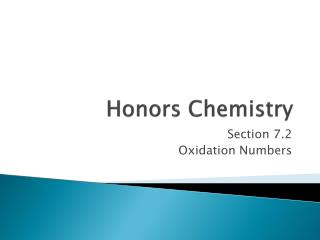DownloadDownload PresentationHonors Chemistry

# Honors Chemistry

Télécharger la présentation## Honors Chemistry

- - - - - - - - - - - - - - - - - - - - - - - - - - - E N D - - - - - - - - - - - - - - - - - - - - - - - - - - -
##### Presentation Transcript

1. Honors Chemistry Section 7.2 Oxidation Numbers

2. Oxidation Numbers • Numbers called oxidation numbers can be assigned to atoms in order to keep track of electron distributions in molecular as well as ionic compounds.

3. Oxidation Numbers • The charges on the ions in an ionic compound reflect the electron distribution of the compound. • In order to indicate the general distribution of electrons among the bonded atoms in a molecular compound or a polyatomic ion, oxidation numbers are assigned to the atoms composing the compound or ion. • Unlike ionic charges, oxidation numbers do not have an exact physical meaning: rather, they serve as useful “bookkeeping” devices to help keep track of electrons. • Oxidation numbers may have unusual values such as fractions.

4. Assigning Oxidation Numbers • In general when assigning oxidation numbers, shared electrons are assumed to “belong” to the more electronegative atom in each bond. • More-specific rules are provided by the following guidelines. • The atoms in a pure element have an oxidation number of zero. examples: all atoms in sodium, Na, oxygen, O2, phosphorus, P4, and sulfur, S8, have oxidation numbers of zero.

5. Assigning Oxidation Numbers • The more-electronegative element in a binary compound is assigned a negative number equal to the charge it would have as an anion. Likewise for the less-electronegative element. • Fluorine has an oxidation number of –1 in all of its compounds because it is the most electronegative element.

6. Assigning Oxidation Numbers • Oxygen usually has an oxidation number of –2. Exceptions: • In peroxides, such as H2O2, oxygen’s oxidation number is –1. • In compounds with fluorine, such as OF2, oxygen’s oxidation number is +2. • Hydrogen has an oxidation number of +1 in all compounds containing elements that are more electronegative than it; it has an oxidation number of –1 with metals.

7. Assigning Oxidation Numbers • The algebraic sum of the oxidation numbers of all atoms in an neutral compound is equal to zero. • The algebraic sum of the oxidation numbers of all atoms in a polyatomic ion is equal to the charge of the ion. • Although rules 1 through 7 apply to covalently bonded atoms, oxidation numbers can also be applied to atoms in ionic compounds similarly.

8. Practice Assign oxidation numbers to each atom in the following compounds or ions: • BF3 • H2S • UF6 • H2SO4

9. Practice Assign oxidation numbers to each atom in the following compounds or ions: • KClO4 • SO32- • NF3 • CO2

10. Using Oxidation Numbers for Formulas and Names • As shown in the table in the next slide, many nonmetals can have more than one oxidation number. • These numbers can sometimes be used in the same manner as ionic charges to determine formulas. • example: What is the formula of a binary compound formed between sulfur and oxygen? From the common +4 and +6 oxidation states of sulfur, you could predict that sulfur might form SO2 or SO3. Both are known compounds.

11. Common Oxidation States of Nonmetals

12. Using Oxidation Numbers for Formulas and Names • Using oxidation numbers, the Stock system, introduced in the previous section for naming ionic compounds, can be used as an alternative to the prefix system for naming binary molecular compounds.

13. Practice • Name the following compound using the Stock System: • CO • CO2